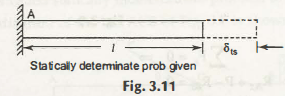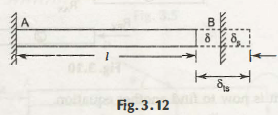The temperature of a body can be increased or decreased by raising or lowering it. Along with decrease and increase in temperature of a body, the dimension also gets affected and increased or decreased. Therefore, increment of stress because of thermal deformations is known as thermal  stresses. On the same way the strains due to this deformation is known as thermal deformation.

It can easily be explained by the following figure as –Now, if the length of the bar is l, and it raised by the temperature Δt,

δts = l σ (Δt)

Here, σ is the coefficient of thermal expansion.

In the case of the following figure

In case of exerting some force which then used to prevent the exact elongation.At this condition

δts = elongation, this takes place due to increase or decrease of temperature M

δts = Shortening of the rod and it takes place due to Force P on the wall

Thus, δts = δs + δ

la Δt = PL / AE + δ.

From this equation the value of P can be easily calculated.

Now, thermal stress cr = -P /A

It will be easier to calculate by providing the exact area of a cross section of the bar A.

Links of Previous Main Topic:-

Links of Next Mechanical Engineering Topics:-

### Submit Your Assignment### Customer Reviews

My Homework Help
Rated 5.0 out of 5 based on 510 customer reviews at